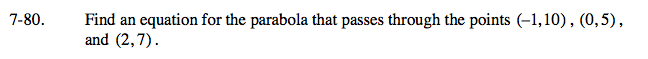Home > A2C > Chapter 7 > Lesson 7.1.5 > Problem7-80

7-80.

Find an equation for the parabola that passes through the points (−1, 10), (0, 5), and (2, 7). 7-80 HW eTool (Desmos). Homework Help ✎Create a system of three equations with three variables using the points and the equation y = ax2 + bx + c.

ab + c = 10
c = 5
4a + 2b + c = 7

Substitute the value for c into the first and third equations to simplify.
You now have a system of two equations with two variables to solve.

y = 2x2 − 3x + 5

Use the eTool below to check your solution graphically.
Click the link at right for the full version of the eTool: A2C 7-80 HW eTool